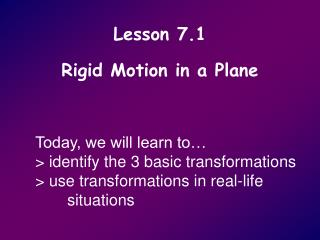DownloadDownload PresentationLesson 7.1 Rigid Motion in a Plane

# Lesson 7.1 Rigid Motion in a Plane

Download Presentation## Lesson 7.1 Rigid Motion in a Plane

- - - - - - - - - - - - - - - - - - - - - - - - - - - E N D - - - - - - - - - - - - - - - - - - - - - - - - - - -
##### Presentation Transcript

1. Lesson 7.1Rigid Motion in a Plane Today, we will learn to… > identify the 3 basic transformations > use transformations in real-life situations

2. Transformations The original figure is called the ____________ and the new figure is called the ____________. preimage image

3. Transformations Preimage: A , B , C , D Image: A’ , B’ , C’ , D’

4. R R REFLECTION

5. R R Rotation

6. R R Translation

7. Isometries preserve length, angle measures, parallel lines, & distances between points

8. Theorems 7.1, 7.2, & 7.4Reflections, translations, and rotations are isometries.

9. 1. Name and describe the transformation. the y-axis reflection over A’B’C’ ABC 

10. 2. Name the coordinates of the vertices of the preimage and image. (0,4) (-4,4) (4,4) (-4,0) (4,0)

11. 3. Name and describe the transformation. x = -1 reflection over HGFE ABCD 

12. 4. Is the transformation an isometry? Explain. NO YES NO YES

13. 5. The mapping is a reflection. Which side should have a length of 7? Explain. WX = 7

14. 6. Name the transformation. Find x and y. Reflection x = 40 y = 4

15. 7. Name the transformation. Find x and y. Reflection x = 12 y = 4

16. 8. Name the transformation. Find a, b, c, and d. reflection a = 73 b = 53 15 c = 8 d =

17. 9. Name the transformation. Find p, q, and r. rotation p = 19 q = 3 r = 7.5

18. 10. Name the transformation and complete this statement GHI   ____ LKP reflection

19. 11. Name the transformation that maps the unshaded turtle onto the shaded turtle reflection translation rotation

20. Lesson 7.2Reflections Today, we will learn to… > identify and use reflections > identify relationships between reflections and line symmetry

21. Reflection 2 images required

22. What is the line of reflection? 1. Is this a reflection? YES x = -2

23. What is the line of reflection? 3. Is this a reflection? YES y = 1

24. What is the line of reflection? 4. Is this a reflection? YES y = x

25. What is the line of reflection? 5. Is this a reflection? YES y = - x

26. When can I use this in “Real Life?” Finding a minimum distanceTelephone Cable - Pole PlacementTV cable (Converter Placement)Walking DistancesHelps you work smarter not harder

27. Finding a minimum distance6. A new telephone pole needs to be placed near the road at point C so that the length of telephone cable (AC + CB) is a minimum distance. Two houses are at positions A and B. Where should you locate the telephone pole?

28. Finding a minimum distance A B C 1) reflect A 2) connect A’ and B A’ 3) mark C

29. GSP

30. Line of Symmetry 1 image reflects onto itself

31. 1 2 3 4 5 6 7 8 7. How many lines of symmetry does the figure have?

32. 180˚ n m A = can be used to calculate the angle between the mirrors in a kaleidoscope n = the number of lines of symmetry

33. 1 2 3 4 5 6 180˚ 8 = 7 8 22.5˚

34. 180˚ 9 = 20˚ http://kaleidoscopeheaven.org

35. 180˚ 4 10. Find the angle needed for the mirrors in this kaleidoscope. = 45˚

36. Project? 1) Identify a reflection in a flag 2) Identify a line of symmetry

37. Reflection Line of Symmetry

38. Reflection Line of Symmetry

39. Section 7.2 Practice Sheet !!!

40. Lesson 7.3Rotations students need tracing paper Today, we will learn to… > identify and use rotations

41. Rotation Direction of Rotation? Center of Rotation? Angle of Rotation?

42. 60˚ 60˚ Angle of Rotation? Clockwise rotation of 60° Center of Rotation?

43. 40° 40° Counter-Clockwise rotation of 40°

44. Theorem 7.3A reflection followed by a reflection is a rotation. If x˚ is the angle formed by the lines of reflection, then the angle of rotation is 2x°.

45. A’ 2x˚ B’ x˚ B’’ A’’ A B

46. A’ A A’’ 1. What is the degree of the rotation? 70˚ 140˚